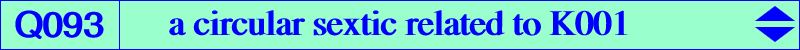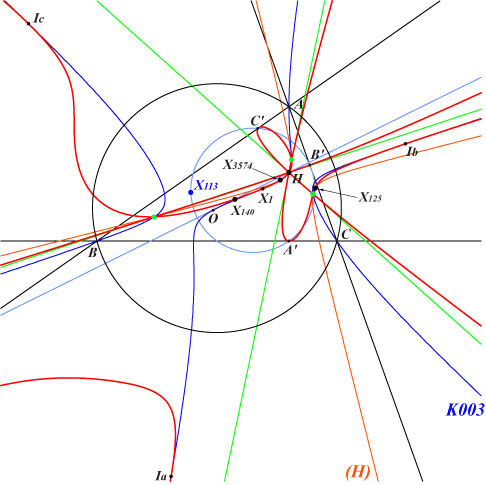too complicated to be written here. Click on the link to download a text file.X(1), X(4), X(30), X(125), X(140), X(3574) excenters A', B', C' vertices of the orthic triangle infinite points of K005 green points described belowDenote by Ap, Bp, Cp the pedal triangle of P. The Euler lines of triangles – PBpCp, PCpAp, PApBp concur (at Q) if and only if P lies on the Neuberg cubic (together with the line at infinity), – ABpCp, BCpAp, CApBp concur (at Q) if and only if P lies on the Napoleon cubic (together with the line at infinity). In both cases, the locus of Q is the circular sextic Q093 with focus X(113). H is a quadruple point on the curve and the three tangents at H are parallel to the asymptotes of K003. Each tangent at H meets Q093 at H (four times) and at another double point which also lies on K003, hence this double point is the O-Ceva conjugate of one infinite point of K003, on the bicevian conic C(G, O) with center X(140), passing through X(125), X(1511), X(2972), since this conic is the O-Ceva conjugate of the line at infinity, on the rectangular hyperbola (H) passing through X(4), X(5), X(125), X(1352), X(2574), X(2575) which is the homothetic of the Jerabek hyperbola under h(H,1/2), on the circle passing through X(1511) whose center is the midpoint of X(5)X(2883). See the paragraph "parallels to the asymptotes of K003" in the page K003. These three double points are represented in green on the figure. Q093 is tangent at the in/excenters to K003 thus the tangents at these points pass through O.